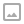## 「模板」陌上花开-CDQ分治+树状数组

·  ✏️ About  1469 words  ·  ☕ 3 mins read · 👀... views

Luogu P3810

## 代码

  1 2 3 4 5 6 7 8 9 10 11 12 13 14 15 16 17 18 19 20 21 22 23 24 25 26 27 28 29 30 31 32 33 34 35 36 37 38 39 40 41 42 43 44 45 46 47 48 49 50 51 52 53 54 55 56 57 58 59 60 61 62 63 64 65 66 67 68 69 70 71 72 73 74 75 76 77 78 79 80 81 82 83 84 85 86 87 88 89 90 91 92 93 94 95 96 97 98 99 100 101 102 103 104 105 106 107 108 109 110  #include using namespace std; int n,k; const int MAXN = 210000; namespace fast_io{ //... }using namespace fast_io; namespace BIT{ int sumn[MAXN]; int lowbit(int x){return x & (-x);} void add(int x,int d){ for(;x <= k;x += lowbit(x)) sumn[x] += d; } int query(int x){ int ans = 0; for(;x >= 1;x -= lowbit(x)) ans += sumn[x]; return ans; } } struct Q{ int a,b,c; Q(){} Q(int x,int y,int z):a(x),b(y),c(z){} bool operator < (Q w)const{ if(a != w.a) return a < w.a; if(b != w.b) return b < w.b; else return c < w.c; } bool operator == (Q w)const{ return a == w.a && b == w.b && c == w.c; } }q[MAXN]; bool judge(int x,int y){ // 用于第二维的归并判断 if(q[x].b!=q[y].b) return q[x].b < q[y].b;//比较两数的c if(q[x].c!=q[y].c) return q[x].c < q[y].c;//比较两数的c else return x < y;//最后比较两数的id } int d[MAXN],ans[MAXN],tt[MAXN]; int tmp[MAXN]; int tot,l,r; void CDQ(int *t,int num,int depth = 0){ //t -> t[num-1] (num个元素） if(num == 1) return; int mid = num/2; CDQ(t,mid,depth+1),CDQ(t+mid,num-mid,depth+1); // 递归分治问题 for(tot = 0,l = 0,r = mid;l < mid && r < num;tot++){ //归并过程，统计左半区间对右半区间的贡献 //如果在左区间，就把其当作修改，更新树状数组 //如果在右区间，就把其当作查询，查询树状数组，更新答案 if(judge(t[l],t[r])) BIT::add(q[t[l]].c,1),tmp[tot] = t[l++]; else ans[t[r]] += BIT::query(q[t[r]].c),tmp[tot] = t[r++]; } //剩余的归并 while(l < mid) BIT::add(q[t[l]].c,1),tmp[tot++] = t[l++]; while(r < num) ans[t[r]] += BIT::query(q[t[r]].c),tmp[tot++] = t[r++]; for(int i = 0;i=1;--i){ // 累计相同元素的贡献 if(q[i] == q[i+1]) ans[i] = ans[i+1] + 1; tt[i] = i; } CDQ(tt+1,n); for(int i = 1;i<=n;i++) ++d[ans[i]]; for(int i = 0;iWRITTEN BY
cqqqwq
A student in Computer Science.